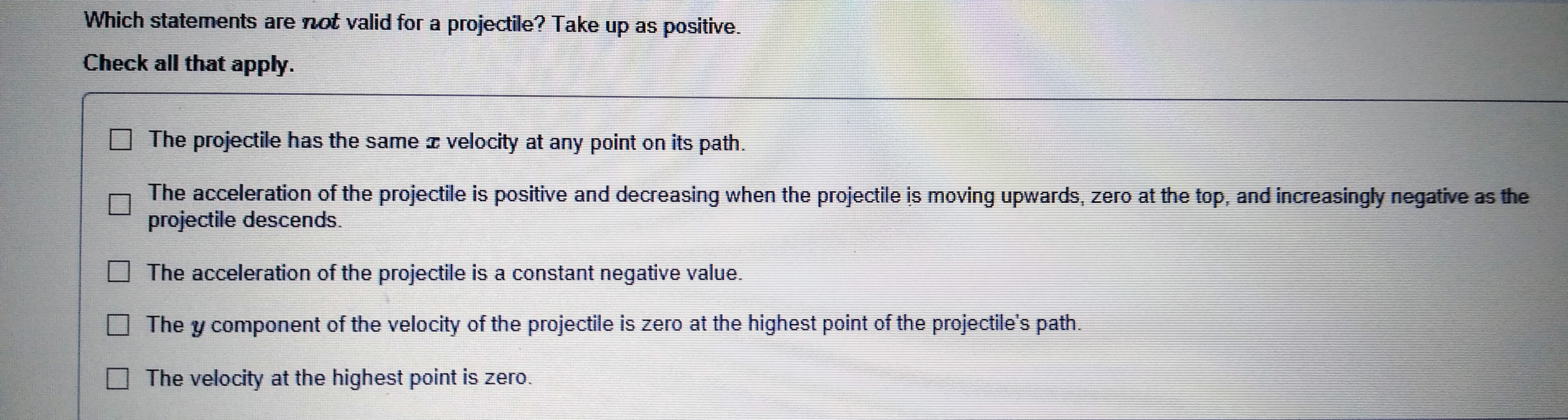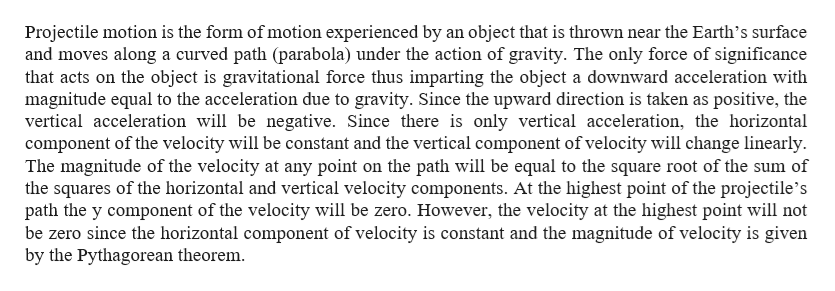# Which statements are not valid for a projectile? Take up as positive.Check all that apply.The projectile has the samevelocity at arny point on its path.The acceleration of the projectile is positive and decreasing when the projectile is moving upwards, zero at the top, and increasingly negative as theprojectile descends.The acceleration of the projectile is a constant negative value.The y component of the velocity of the projectile is zero at the highest point of the projectile's pathThe velocity at the highest point is zero

Question
74 viewshelp_outlineImage TranscriptioncloseWhich statements are not valid for a projectile? Take up as positive. Check all that apply. The projectile has the same velocity at arny point on its path. The acceleration of the projectile is positive and decreasing when the projectile is moving upwards, zero at the top, and increasingly negative as the projectile descends. The acceleration of the projectile is a constant negative value. The y component of the velocity of the projectile is zero at the highest point of the projectile's path The velocity at the highest point is zero fullscreen
check_circle

Step 1help_outlineImage TranscriptioncloseProjectile motion is the form of motion experienced by an object that is thrown near the Earth's surface and moves along a curved path (parabola) under the action of gravity. The only force of significance that acts on the object is gravitational force thus imparting the object a downward acceleration with magnitude equal to the acceleration due to gravity. Since the upward direction is taken as positive, the vertical acceleration will be negative. Since there is only vertical acceleration, the horizontal component of the velocity will be constant and the vertical component ofvelocity will change linearly The magnitude of the velocity at any point on the path will be equal to the square root of the sum of the squares of the horizontal and vertical velocity components. At the highest point of the projectile's path the y component of the velocity will be zero. However, the velocity at the highest point will not be zero since the horizontal component of velocity is constant and the magnitude of velocity is given by the Pythagorean theorem fullscreen

### Want to see the full answer?

See Solution

#### Want to see this answer and more?

Solutions are written by subject experts who are available 24/7. Questions are typically answered within 1 hour.*

See Solution
*Response times may vary by subject and question.
Tagged in

### Physics